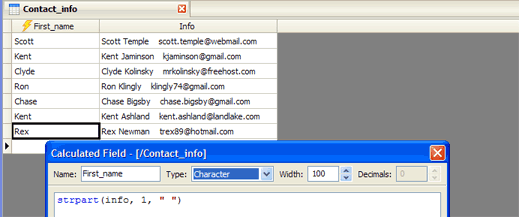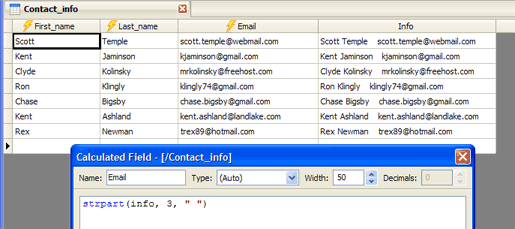Exploring Calculated Fields: Part 2 - Extracting Data Values with STRPART() | Kirix Strata Blog

# Kirix Strata Blog

## Exploring Calculated Fields: Part 2 - Extracting Data Values with STRPART()

In part 1 of this series, we looked at the basics of using calculated fields, including referencing fields and using functions.

In this installment, we'll look at how you can use calculated fields to extract data values from a column with “dirty” data. This happens more than one might think: if you've ever "cleaned" a list of contact information or migrated databases, you know that you often have to take a column and break it up into multiple parts. Calculated fields make this task very easy.

Let's look at an example. Suppose we have a table that contains raw contact information smashed together in a single field:Let's extract each of these parts — first name, last name and email address — from the single column into multiple columns. We'll do this by using a calculated field with a function that parses the string, based on a delimiter (such as a space), and returns the relevant portion of the string.

So we'll go ahead and create a calculated field called “First_name”, having a character type, a width of 100. Using a character type indicates that we our final field should store character information. Using a width of 100 allows this field to store up to 100 characters, which should be enough for this example.

The formula itself uses the STRPART() function, short for "String Part.” String Part takes a field, the “part” of the field you want and a delimiter, if any:

`STRPART(string, section number [, list])`

So, in this case, we'll parse the field “info”, taking the first part of it an using a space as a delimiter:

`strpart(info, 1, " ")`

As we see, this creates a field that extracts out the first part of the info field, which in this case, is the first name:In the same way, we can extract out the other values in the table. We just need to create two more calculated fields using the same pattern, one for the last name and the other with the email.

So, for the second calculated field, "Last_name," we create a calculated field with the following formula:

`strpart(info, 2, " ")`

For the third calculated field, "Email", we use the following formula:

`strpart(info, 3, " ")`

Really, the only difference here is that the formula is different; in the second and third formulas, we extract the second and third part of the string, hence the "2" and the "3" in the formulas.

Once we've done this, we see the following…At this point, we can leave these calculated fields and continue with our analysis or we can easily convert these to “normal” fields by right-clicking on the column header and selecting "Convert to Fixed Field(s)" from the right-click menu.

Of course, data is rarely ever this "clean", so sometimes we need more advanced functions for extracting out values from columns. One of the most powerful of these function is REGEXP(), which is a function for extracting values using regular expressions. In the third part of this series, we'll take a look at how to use regular expressions to extract values.

### 3 Responses to “Exploring Calculated Fields: Part 2 - Extracting Data Values with STRPART()”

1. Exploring Calculated Fields: Part I - The Basics | Strata Blog says:

[…] Blog « Using Relationships to Compare Lists of Emails Exploring Calculated Fields: Part 2 - Extracting Data Values with STRPART() […]

2. JohnDoe says:

How to get the next record's field as part of calculated field? For example, I have a column that calculate average of previous 5 values of another column. Currently, I notice all the calculations are based on current records.

3. Ken Kaczmarek says:

Hi JohnDoe — that is correct, all calculations are based within the same record, so you won't be able to calculate “up or down” your table. Spreadsheets, like Microsoft Excel, are good at dealing with “cells”, since they can be more free-wheeling in their calculations. Unfortunately, databases tend to be constrained much more to individual records (e.g., calculated field) or an entire field (e.g., a query or group), rather than just a subset of a field.